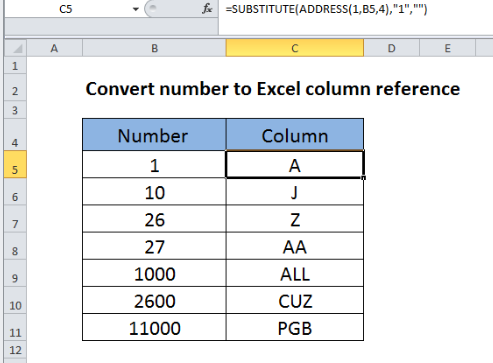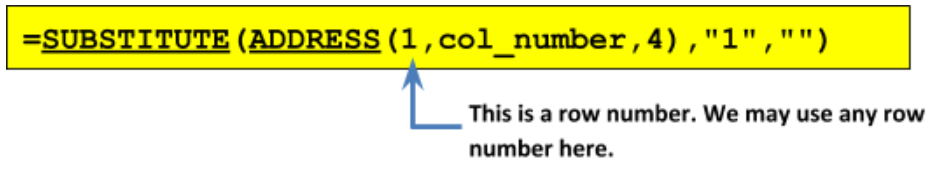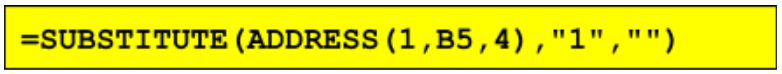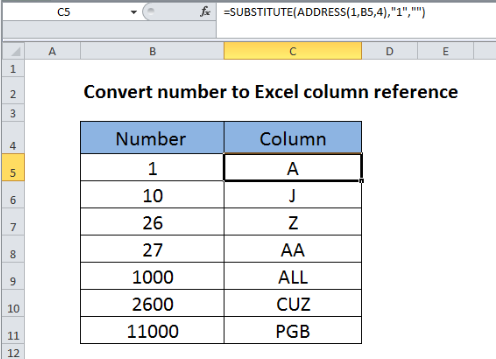Get instant live expert help with Excel or Google Sheets“My Excelchat expert helped me in less than 20 minutes, saving me what would have been 5 hours of work!”

#### Post your problem and you’ll get expert help in seconds.

Your message must be at least 40 characters
Our professional experts are available now. Your privacy is guaranteed.

# How to Convert Column Numbers to Letters in Excel

## Convert column numbers to letters

In this example, we want to input the column number in the first column of the table then allow the second column to show the corresponding Excel column letter. Letter A corresponds to the first column in an Excel worksheet, B to the second column, C to the third column, and so on.Figure 1. Convert column numbers to letters

To convert a column number to an Excel column letter (i.e, A, B, C, etc.) we will make use a formula that utilizes the ADDRESS and SUBSTITUTE functions.

The general formula would beFigure 2. Convert column numbers to letters

In our example, the formula in cell C5 will beFigure 3. Convert column numbers to letters

The ADDRESS function returns a text representation of a cell address. It is a built-in function in Excel that can be used as a look-up function. For example, the formula =ADDRESS(1,1) will return the value \$A\$1. The ADDRESS function can be entered as part of a formula or as a parameter within another function.

The first step is to generate an address that carries the column number. We will do this with the ADDRESS function, by providing 1 for row number (we can use any row number and this will be hard-coded, or fixed, in the formula), a column number from cell B5, and we will use 4 for the abs_num argument to get a relative reference to the cell address. Note that the four possible values for the abs_num argument are: 1 (or omitted) for absolute reference; 2 for absolute row and relative column reference; 3 for relative row and absolute column reference; and 4 for relative reference.

With the information provided, our ADDRESS function will return the text string “A1”. We will then use the SUBSTITUTE function to remove the number portion of the text and retain only the column letter.Figure 4. Convert column numbers to letters

## Using the SUBSTITUTE Function

The SUBSTITUTE function is used to replace specific parts of a text with another by means of matching.  For example, the formula `=SUBSTITUTE(“123-456-789”, “-”, “”)` will return “123456789” because we specify in the formula to replace every occurrence of “-” in the main text with the null string (“”), thereby removing the dashes. Note that the SUBSTITUTE function is case-sensitive.

As mentioned, we will use the SUBSTITUTE function to remove the number portion of the text cell address reference and retain only the column letter. Here is how it’s done: In all cases, we can confidently locate “1” and then replace it with the null string (“”)

i.e., `=``SUBSTITUTE (“A1”,”1”,””)`.

This is because the number on the row is hard-coded as “1” inside the ADDRESS function.

## Instant Connection to an Expert through our Excelchat Service

Most of the time, the problem you will need to solve will be more complex than a simple application of a formula or function. If you want to save hours of research and frustration, try our live Excelchat service! Our Excel Experts are available 24/7 to answer any Excel question you may have. We guarantee a connection within 30 seconds and a customized solution within 20 minutes.

### Did this post not answer your question? Get a solution from connecting with the expert.Another blog reader asked this question today on Excelchat:
Related blogs
Solution examplesI am trying to figure out how to use a sumif on SUBSTITUTE(A2,"*","~*") where A2 is a formula with 3371-4
Solved by M. Y. in 19 minsHello, I need help with IF and SUBSTITUTE formula in excel
Solved by A. A. in 43 mins## Subscribe to Excelchat.coAnother blog reader asked this question today on Excelchat: